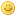Mizugadro, pentation, BookMphLeeLong Time FellowPosts: 374 Threads: 30 Joined: May 2013 02/17/2015, 03:36 PM (02/10/2015, 02:40 PM)Kouznetsov Wrote: I think, you are doing in the correct way. I hope, you made no errors. Keep in mind, that $L$ is fixed point, id est, $b^L=L$I have to notice that I didn't deduced it but just observed the progression of your formulas on your book. Anyways I tried to substitute YOUR solution $\displaystyle F(z) = L+\sum_{n=1}^{\infty} a_n {\varepsilon^n}$ inside the transfer equation $F(z+1)=\exp_b(F(x))$... but my deduction has some holes, I need some algebraic manipulation in order to come at your final form: In fact developing $\exp_b(F(x))$ I get $\displaystyle \exp_b(F(x))= \exp_b( L+\sum_{n=1}^{\infty} a_n {\varepsilon^n} )=\sum_{i=0}^{\infty}{\ln(b)^i\over i!}\left ( L+\sum_{n=1}^{\infty} a_n {\varepsilon^n} \right)^i$ that looks very different from the series you show in your book at formula (6.4) $\displaystyle \exp_b(F(x))=L+\sum_{i=0}^{\infty}{T^{[i]}(L)\over i!}\left ( L+\sum_{n=1}^{\infty} a_n {\varepsilon^n} \right)^i=L+\sum_{i=0}^{\infty}{\ln(b)^i b^L\over i!}\left (\sum_{n=1}^{\infty} a_n {\varepsilon^n} \right)^i$ (6.4) So the question is how one can go from $\displaystyle\sum_{i=0}^{\infty}{\ln(b)^i\over i!}\left ( L+\sum_{n=1}^{\infty} a_n {\varepsilon^n} \right)^i$ TO $L+\sum_{i=0}^{\infty}{\ln(b)^i b^L\over i!}\left (\sum_{n=1}^{\infty} a_n {\varepsilon^n} \right)^i$? And how you can manipulate the series $L+\sum_{i=0}^{\infty}{\ln(b)^i b^L\over i!}\left (\sum_{n=1}^{\infty} a_n {\varepsilon^n} \right)^i$ and obtain a series of the form $L+\sum_{n=1}^{\infty} \tau_n {\varepsilon^n}$? In other words Quote:Do you have any software at your computer to check your deduction? I used Maple; then I found that it is not so good, http://en.wikisource.org/wiki/Maple_and_Tea and Mathematica does this better. In addition, I use C++ to plot graphics and the complex maps, and you can do the same. You may use my algorithms and codes, and you may write your own codes, it is better for your education. Keep in mind, that all codes have bugs. If you get some tens coefficients with Mathematica and use them in the C++ code, the resulting implementation may return of order of 14 significant figures, and allow to plot the complex maps in the real time. You may load the examples from http://mizugadro.mydns.jp/t/index.php/Category:Book http://mizugadro.mydns.jp/t/index.php/Category:C%2B%2B Try to write your own algorithms. Run the tests. Substitute the functions you get into the equations they are supposed to satisfy. Plot the residuals. Compare your calculus to my calculus. Try to make your algorithms more precise, than my ones. Try to make your algorithms shorter and simpler, than my ones. Try to make your algorithms faster, than my ones. Try to make your algorithms more general, than my ones. Citius, Altius, Fortius! No I don't have powerful softwood and I don't even know how C++ works (I'd need a massive amount of time to learn it) ------------------ Other topic: With your supefunction formalism/metods/techniques(or theory or how you call it) is possible to find solution to factorial-like problems where there are involved families of different functions instead of only one function iterated many times? Consider this general problem Given a family of functions $f_0,f_1,f_2,...$ we define a function $H$ such that it satisfies this equations $H(0)=\beta$ $H(n+1)=f_n(H(n))$ Is it possible to find an unique extensions of $H$ to the real/complex numbers? This is a very general problem: if$f_n(x)=n\cdot x$ and $\beta=1$ then $H(n)=n!$ and its extension is the gamma function but we can still achieve uniqueness for other sequences $f_0,f_1,f_2,...$ of functions? Thank you again for the kindness! MSE MphLee Mother Law $(\sigma+1)0=\sigma (\sigma+1)$ S Law $\bigcirc_f^{\lambda}\square_f^{\lambda^+}(g)=\square_g^{\lambda}\bigcirc_g^{\lambda^+}(f)$sheldonisonLong Time FellowPosts: 684 Threads: 24 Joined: Oct 2008 03/02/2015, 08:13 PM (This post was last modified: 03/02/2015, 08:16 PM by sheldonison.) How many sample points are required for the Kouznetsov Cauchy integral method to get double precision (51 bits=16 decimal digits) accuracy? I seem to remember it was 1000-2000 sample points; also, how many iterations? Do you have any estimates on how fast the number of sample points required grows? For example, what it would take to get twice that precision, 32 decimal digits? - Sheldon « Next Oldest | Next Newest »

 Possibly Related Threads… Thread Author Replies Views Last Post Pentation fractal Daniel 11 1,672 08/03/2022, 03:04 AM Last Post: JmsNxn pentation and hexation sheldonison 9 23,160 09/18/2019, 02:34 PM Last Post: sheldonison Tetration is pentation. This deserve more thinking. marraco 2 7,589 03/30/2015, 02:54 PM Last Post: marraco Infinite Pentation (and x-srt-x) andydude 20 49,395 05/31/2011, 10:29 PM Last Post: bo198214 Regular "pentation"? mike3 12 36,728 04/04/2011, 03:16 AM Last Post: BenStandevenPentation roots self but please you do... nuninho1980 2 11,359 11/03/2010, 12:54 PM Last Post: nuninho1980 book: the theory of fractional powers of operators bo198214 2 10,011 06/22/2010, 07:30 PM Last Post: Ztolk Pentation's definitional ambiguity Base-Acid Tetration 14 39,528 12/15/2009, 11:23 PM Last Post: Base-Acid Tetration Complex fixed points of base-e tetration/tetralogarithm -> base-e pentation Base-Acid Tetration 19 54,372 10/24/2009, 04:12 AM Last Post: andydude online-book: Milnor, dynamics in one complex variable bo198214 0 7,635 05/23/2009, 04:21 PM Last Post: bo198214

Users browsing this thread: 1 Guest(s)